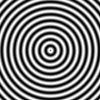#### 4.3Conversion and Construction

 procedure(flomap->bitmap fm) → Any fm : flomap
Converts a flomap to a bitmap%.

The return type is imprecise because Typed Racket does not support the object system well yet. As a typed function, this is most useful in DrRacket’s REPL to visualize flomaps; any other typed use is difficult.

Flomaps are interpreted differently depending on the number of components:
• Zero components. Raises an error.

• One component. Interpreted as intensity (grayscale).

• Two components. Interpreted as AL, or alpha+intensity, with intensity multiplied by alpha.

• Three components. Interpreted as RGB.

• Four components. Interpreted as ARGB with color components multiplied by alpha.

• More components. Raises an error.

See Opacity (Alpha Components) for a discussion of opacity (alpha) representation.

A zero-size fm is padded by one point in any zero direction before conversion. For example, if fm is size 0×1, the result of (flomap->bitmap fm) is size 1×1.

Values are clamped to between 0.0 and 1.0 before conversion.

 procedure(bitmap->flomap bm) → flomap bm : Any
Given a bitmap% instance, returns an ARGB flomap with alpha-multiplied color components. See Opacity (Alpha Components) for a discussion of opacity (alpha) representation.

The argument type is imprecise because Typed Racket does not support the object system well yet.

 procedure(make-flomap c w h [v]) → flomap c : Integer w : Integer h : Integer v : Real = 0.0
Returns a w×h flomap with c components, with every value initialized to v. Analogous to make-vector.

To create flomaps filled with a solid color, use make-flomap*.

 procedure(make-flomap* w h vs) → flomap w : Integer h : Integer vs : (U (Vectorof Real) FlVector)
Returns a w×h flomap with each point’s components initialized using the values in vs. Analogous to make-vector.

The following two examples create an RGB and an ARGB flomap:
 > (flomap->bitmap (make-flomap* 100 100 #(0.5 0.0 1.0)))> (flomap->bitmap (make-flomap* 100 100 #(0.5 0.25 0.0 0.5)))See Opacity (Alpha Components) for a discussion of opacity (alpha) representation.

 procedure(build-flomap c w h f) → flomap c : Integer w : Integer h : Integer f : (Nonnegative-Fixnum Nonnegative-Fixnum Nonnegative-Fixnum -> Real)
Returns a w×h flomap with c color components, with values defined by f. Analogous to build-vector.

The function f receives three arguments k x y: the color component and two positional coordinates.

Examples:

 > (flomap->bitmap (build-flomap 1 100 100 (λ (k x y) (/ (+ x y) 200))))> (define sine-fm (build-flomap 1 100 100 (λ (k x y) (* 1/2 (+ 1 (sin (sqrt (+ (sqr (- x 50)) (sqr (- y 50))))))))))
> (flomap->bitmap sine-fm)To build a flomap using a function that returns vectors, see build-flomap*.

procedure

(build-flomap* c w h f)  flomap

c : Integer
w : Integer
h : Integer
f :
 (Nonnegative-Fixnum Nonnegative-Fixnum -> (U (Vectorof Real) FlVector))
Returns a w×h flomap with c color components. Its values are defined by f, which returns vectors of point components. The vectors returned by f must be length c.

Analogous to build-vector.

Examples:

 > (flomap->bitmap (build-flomap* 4 100 100 (λ (x y) (vector (/ (+ x y) 200) (/ (+ (- 100 x) y) 200) (/ (+ (- 100 x) (- 100 y)) 200) (/ (+ x (- 100 y)) 200)))))> (build-flomap* 4 100 100 (λ (x y) (vector (/ (+ x y) 200))))

build-flomap*: expected argument of type <length-4 Vector or

FlVector>; given: '#(0)

 procedure(draw-flomap draw w h) → flomap draw : (Any -> Any) w : Integer h : Integer
Returns a w×h bitmap drawn by draw. Analogous to slideshow’s dc.

The draw function should accept a dc<%> instance and use its drawing methods to draw on an underlying bitmap. The bitmap is converted to a flomap using bitmap->flomap and returned. See Floating-Point Bitmaps for an example.

This function is very difficult to use in Typed Racket, requiring occurrence checks for, and use of, experimental types. However, as Typed Racket grows to handle Racket’s object system, the types will be made more precise.

 procedure fm : flomap
 procedure fm : flomap
Multiplies/divides each nonzero-component value with the corresponding zero-component value. Dividing by 0.0 produces 0.0.

In other words, flomap-multiply-alpha converts non-alpha-multiplied flomaps into alpha-multiplied flomaps, and flomap-divide-alpha converts them back.

You should not generally have to use these functions, because bitmap->flomap returns an alpha-multiplied flomap and every alpha-aware flomap function assumes its arguments are alpha-multiplied and produces alpha-multiplied flomaps.

See Opacity (Alpha Components) for a discussion of opacity (alpha) representation.

syntax

(inline-build-flomap c w h f)

 c : Integer
 w : Integer
 h : Integer
f :
 (Nonnegative-Fixnum Nonnegative-Fixnum Nonnegative-Fixnum Nonnegative-Fixnum -> Float)
A macro version of build-flomap.

There are three differences between the function f passed to build-flomap and the f passed to inline-build-flomap. First, the f passed to inline-build-flomap can be a macro. Second, it receives arguments k x y i, where i is a precalculated index into the result’s values. Third, it must return a Float.

Using inline-build-flomap instead of build-flomap may ensure that f is inlined, and therefore floats remain unboxed. Many library functions use inline-build-flomap internally for speed, notably fm+ and the other pointwise arithmetic operators.

This is not available in untyped Racket.

syntax

(inline-build-flomap* c w h f)

 c : Integer
 w : Integer
 h : Integer
f :
 (Nonnegative-Fixnum Nonnegative-Fixnum Nonnegative-Fixnum -> FlVector)
A macro version of build-flomap*.

There are three differences between the function f passed to build-flomap* and the f passed to inline-build-flomap*. First, the f passed to inline-build-flomap* can be a macro. Second, it receives arguments x y i, where i is a precalculated index into the result’s values. Third, it must return a FlVector.

This is not available in untyped Racket.